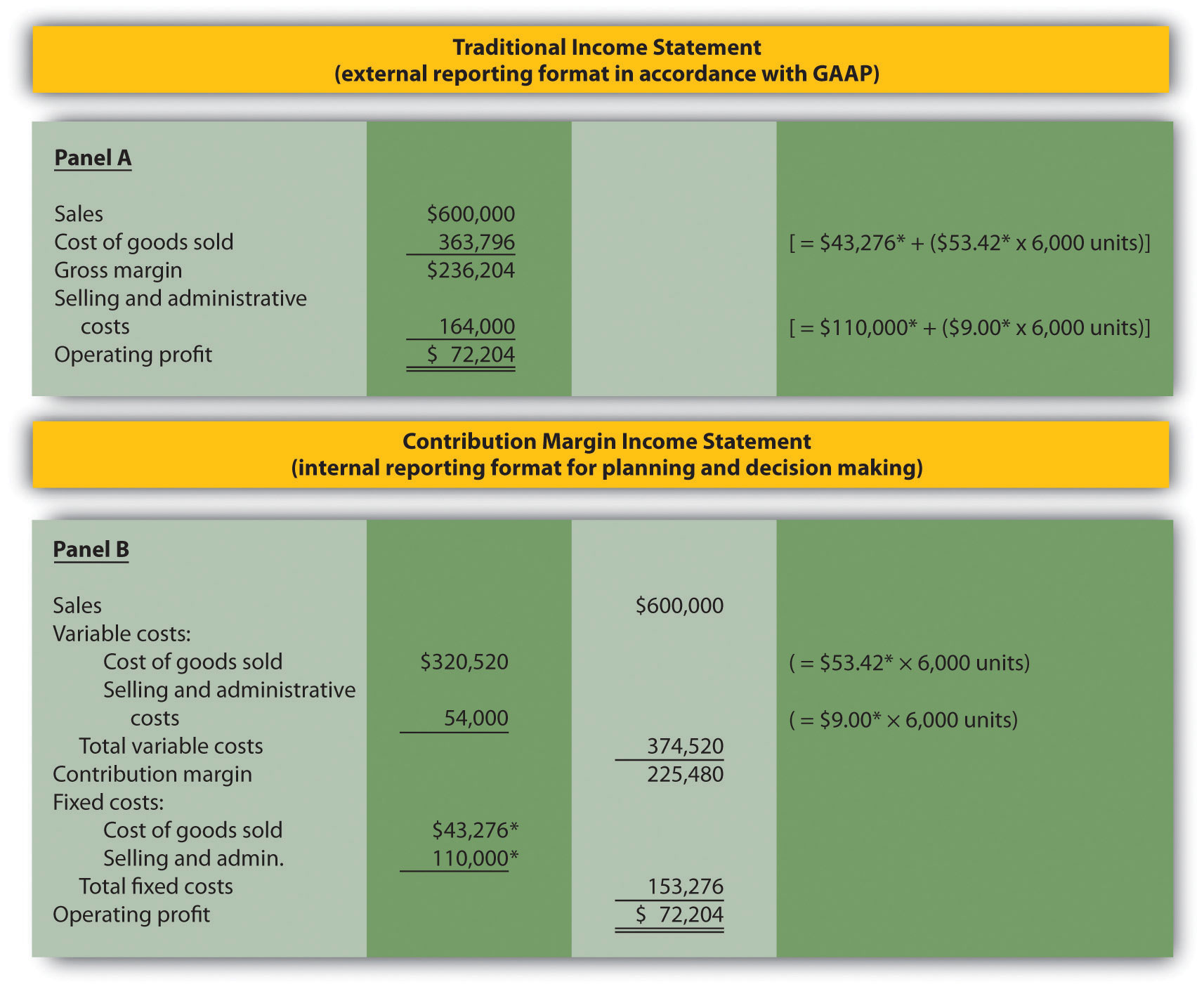## Unit 3 Study Guide: Short-Term Decision Making

### 3a: Identify typical cost behavior patterns.

To predict future profitability, manufacturers identify costs that depend on the number of units manufactured. For example, the manufacture of one table requires the cost of four table legs, whereas the manufacture of two tables requires the cost of eight table legs.

• Define cost behavior pattern.
• Name three cost behavior patterns that can affect whether a company is profitable.

Cost behaviors that change according to different levels of activity are called variable costs.

• Define variable costs.
• Name three of the most common variable costs.
• Identify examples of activities that are useful for estimating variable costs.

Review these definitions in Cost Behavior Patterns from Managerial Accounting. Table 5.1 Variable Cost Behavior for Bikes Unlimited offers an example of variable cost behavior at different levels of activity.

A cost that remains the same regardless of the level of activity is called a fixed cost.

• Define fixed costs.
• Name the two of the most common fixed costs. How are the same and how do they differ?
• Explain the effect of fixed costs on per-unit costs at different levels of production.

Review these definitions in Cost Behavior Patterns from Managerial Accounting. Table 5.2 Fixed Cost Behavior for Bikes Unlimited shows the effect of fixed costs on per-unit fixed costs at different levels of activity.

Mixed costs combine components of both fixed and variable costs.

• Define mixed costs.
• Describe the elements of the mixed cost equation where Y = f + vX
(Y = total mixed costs, f = total fixed costs, v = variable cost per unit, and X = level of activity)

Review these definitions in Cost Behavior Patterns from Managerial Accounting. Table 5.3 Mixed Cost Behavior for Bikes Unlimited shows the effect of mixed costs on per-unit costs at different levels of activity.

Estimating costs involves taking short-term considerations into account, within a relevant range.

• Explain why short-term decision making is important.
• Explain the significance of using a relevant range when considering levels of activity.

Review these considerations in Cost Behavior Patterns from Managerial Accounting.

### 3b: Estimate costs using account analysis, the high-low method, the scattergraph method, and regression analysis.

Organizations use four different methods for estimating fixed and variable costs.

• Define the following methods for estimating fixed and variable costs:
1. Account analysis method;
2. High-low method (four-step process);
3. Scattergraph method (five-step process); and
4. Regression analysis method.
• Describe the strengths and weaknesses of each method.
• Identify the method that is usually the most accurate.

Review these cost estimation methods in Cost Estimation Methods from Managerial Accounting.

Review the following videos:

### 3c: Prepare a contribution margin income statement.

A contribution margin income statement organizes the fixed and variable cost components of a regular income statement.

• How do accountants calculate contribution margin?
• How do accountants calculate operating profit.
• What is the benefit of a contribution margin income statement?

Review these definitions in The Contribution Margin Income Statement from Managerial Accounting.

Review Figure 5.7 which presents an example of a contribution margin income statement.

Figure 5.7 Traditional and Contribution Margin Income Statements for Bikes Unlimited### 3d: Describe the assumptions used to estimate costs.

• List two assumptions managers make when they estimate costs.

Review The Relevant Range and Nonlinear Costs from Managerial Accounting.

### 3e: Perform regression analysis using Excel.

• How do accountants use Excel to perform a regression analysis to estimate fixed and variable costs.

Review the four-step process of performing regression analysis in Appendix: Performing Regression Analysis with Excel from Managerial Accounting.

### Unit 3 Vocabulary

• Account analysis
• Committed fixed cost
• Contribution margin
• Contribution margin income statement
• Cost equation
• Discretionary fixed cost
• Fixed cost
• High-low method
• Income statement
• Mixed cost
• Regression analysis
• Relevant range
• Scattergraph method
• Variable cost